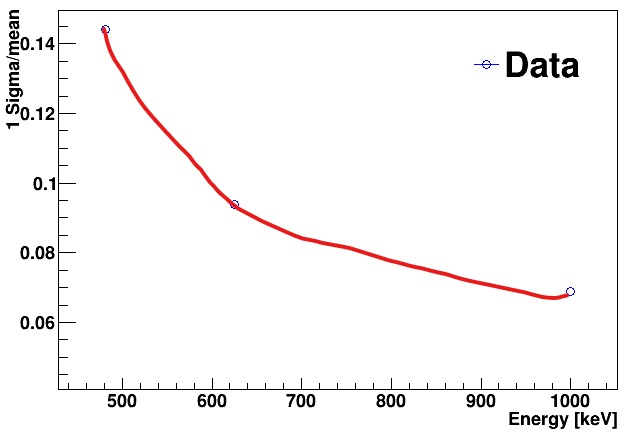Hello Rooters.

I drew the following picture.I want to fit like a blue line and I want to know the function to get as a result of the fitting.

Through 3 points many functions can be used. It is up to you to decide which one is the most
appropriate for you particular problem.

``````TF1 *f= new TF1("f","TMath::Sqrt(  TMath::Pow( ,2) + TMath::Pow(/TMath::Sqrt(x) ,2) +   TMath::Pow(/x ,2)     )")